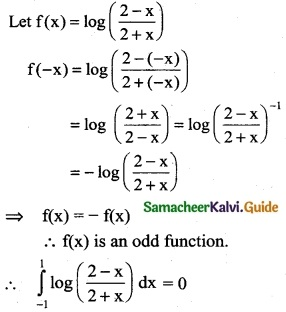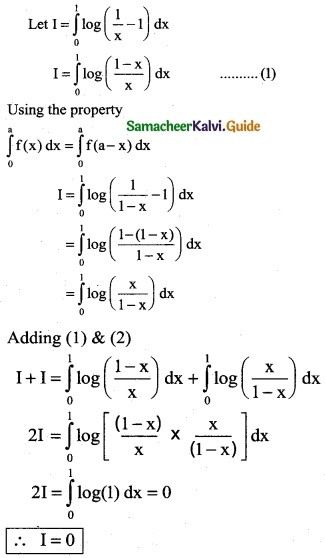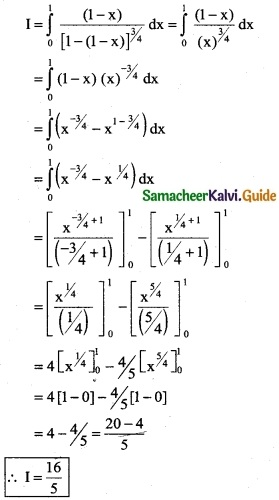Tamilnadu State Board New Syllabus Samacheer Kalvi 12th Business Maths Guide Pdf Chapter 2 Integral Calculus I Ex 2.9 Text Book Back Questions and Answers, Notes.

## Tamilnadu Samacheer Kalvi 12th Business Maths Solutions Chapter 2 Integral Calculus I Ex 2.9

Evaluate the following using properties of definite integrals:

Question 1.
$$\int_{-π/4}^{π/4}$$ x³ cos³ x dx
Solution:
Let f(x) = x³cos³x
f(-x) = (-x)³ cos³(-x)
= -x³ cos³x
f(-x) = -f(x)
⇒ f(x) is an odd function
∴ $$\int_{-π/4}^{π/4}$$ x³ cos³ x dx = 0Question 2.
$$\int_{-π/2}^{π/2}$$ sin² θ dθ
Solution:
Let f(θ)= sin² θ
f(-θ) = sin² (-θ) = [sin (-θ)]²
= [-sin θ]² = sin² θ
f(-θ) = f(θ)
∴ f(θ) is an even functionQuestion 3.
$$\int_{-1}^{1}$$ log($$\frac { 2-x }{2+x}$$) dx
Solution:Question 4.
$$\int_{0}^{π/2}$$ $$\frac { sin^7x }{sin^7x+cos^7x}$$ dx
Solution:
Using the property
$$\int_{0}^{a}$$ f(x) dx = $$\int_{0}^{a}$$ f(a – x) dxQuestion 5.
$$\int_{0}^{1}$$ log ($$\frac { 1 }{x}$$ – 1) dx
Solution:Question 6.
$$\int_{0}^{1}$$ $$\frac { x }{(1-x)^{3/4}}$$ dx
Solution:
Let I = $$\int_{0}^{1}$$ log $$\frac { x }{(1-x)^{3/4}}$$ dx
Using the property
$$\int_{0}^{a}$$ f(x) dx = $$\int_{0}^{a}$$ f(a – x) dx